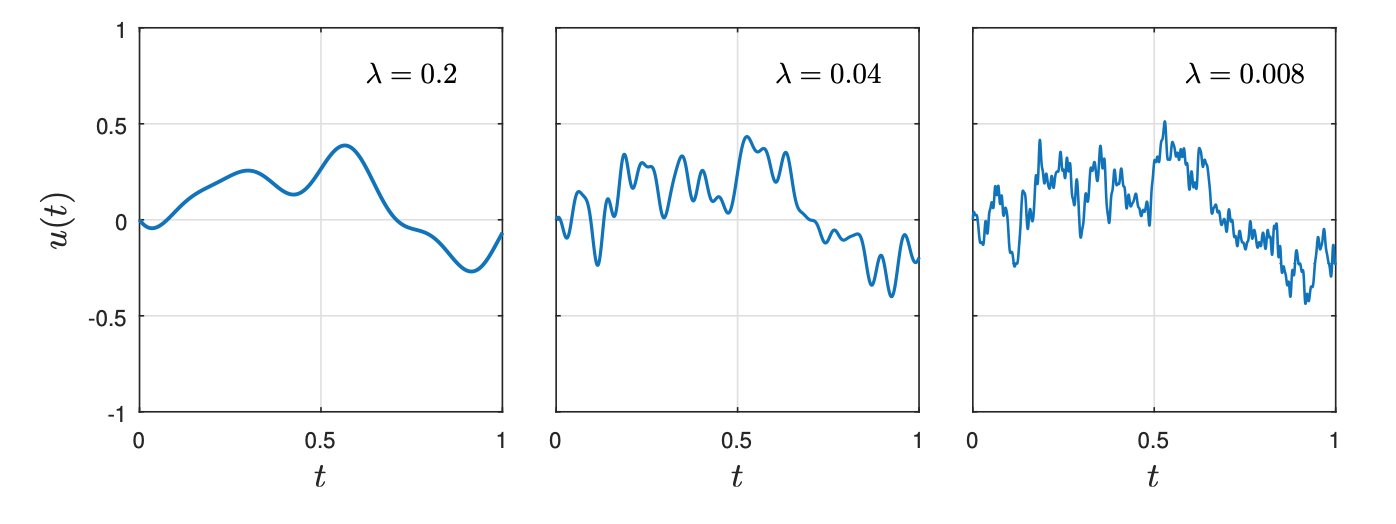# Smooth functions that resemble random walks

If the Riemann hypothesis holds, then the Mertens function $$M(n)\equiv\sum_{x\leq n} \mu(n)$$ behaves much like a 1D random walk. This includes the statements that

1. $$M(n)$$ changes sign infinitely often
2. $$M(n)=O(\sqrt{n})$$ (ignoring subleading logarithmic corrections).

It is also believed that 3) $$\mu(n)=M(n)-M(n-1)$$ "looks random". This seems to be a topic of current research, but is sometimes phrased as the "Mobius randomness law" (Eq 5 here), which says that for functions of low complexity $$\xi (n)$$

$$\sum_{n\leq N} \xi(n) \mu(n) = o(\sum_{n\leq N} |\xi(n)|)$$

Some weaker analogue of this conjecture is proved in the linked note.

Now, the Mertens function can be extended to the reals through an integral expression

$$M(x) = \int^{c+\mathrm{i}\infty}_{c-\mathrm{i}\infty } \frac{ds}{2\pi \mathrm{i}} \, \frac{x^s}{s \zeta(s)}\,\,\,\,\, (*)$$

My question is: Does anyone know of a function $$f:\mathbb{R}\rightarrow \mathbb{R}$$, which is a deterministic$$\dagger$$ combination of known analytic functions (e.g., Eq. $$(*)$$) and which can also be proved to obey conditions 1., 2., and 3. above? Here 3. would mean that $$f(n)-f(n-1)$$ "looks random" in some sense, e.g., the sense described above. Perhaps there are many examples; if so, what's the simplest? I don't particularly care whether $$f(n)$$ takes integer values; I just want it to look like a random walk.

**EDITS/ I've updated the wording of this question. There is an obvious set of examples if I merely insist on 1. and 2. I should have emphasized the need for $$f(n)-f(n-1)$$ to "look random".

$$\dagger=$$ I want $$f$$ to be expressible as a deterministic combination of known functions; so I won't accept e.g., a fourier series with randomly chosen coefficients (see Carlo's answer below). Morally, I'm interested in the appearance of randomness from seemingly deterministic expressions (e.g., $$(*)$$).

• Well, for example $\sqrt{n}\sin n$. In general it’s not useful to ask for soft-analytic properties like smoothness, since for any function from the integers to the reals, there is a smooth function that extends the domain to the reals. Aug 2, 2020 at 16:18
• @user36212 Does $\sqrt{n} \sin n$ obey (1)? Even if you add floor functions I'd be surprised if that's known (or even true). Aug 2, 2020 at 18:11
• Thank you both for your comments. What I really want is for $f(x)$ to "look like a random walk" at integer points. This will certainly require that 1 and 2 are satisfied. However, I obviously need to add an extra condition; something along the lines that $f(n+1)-f(n)$ and $f(m+2)-f(m+1)$ are uncorrelated i.e., steps at different times are uncorrelated. Aug 2, 2020 at 19:48
• Marcus M - no, I presume it doesn’t go through integers at integer values; but that you can fix by smoothly transforming $n$. Point is it looks nothing like a random walk. Aug 2, 2020 at 22:15
• If your interest is mainly in understanding the appearance of randomness (or something that 'looks like it') from compact deterministic expressions, then wouldn't you have more luck looking at pseudo-random number generators than anything to do with analytic functions? Aug 3, 2020 at 11:54

Smooth random functions, random ODEs, and Gaussian processes (2018) describes an approach that takes a finite Fourier series on the interval $$(0,1)$$ with randomly chosen coefficients. The integral of this function approaches Brownian motion in the limit that the number $$M$$ of Fourier coefficients tends to infinity.

The plot shows three such functions, for $$M=1/\lambda=5,25,$$ and $$125$$.For $$M=1000$$ the curve is a Brownian path within plotting accuracy, the plot below shows 10 realizations.• Carlo: Thank you for the example. It's not quite what I was looking for, although interesting. Your answer has led me to refine my question. In particular, I'd like $f(x)$ to have an expression as a deterministic combination of known functions. In your example, it seems to be important that the fourier coefficients are chosen randomly. Aug 3, 2020 at 7:54
• I'm not sure that I understand your point: this is a fully deterministic construction, the Fourier coefficients are just a fixed string of numbers, how I arrived at them should not matter. If you wish, you can construct the coefficients from the digits of $\pi$ --- wouldn't that satisfy your requirement of "appearance of randomness from seemingly deterministic expressions"? Aug 3, 2020 at 8:32
• Hi Carlo, the construction using the digits of pi almost certainly works. It's not quite as compact as $(*)$ but I'll grant that it satisfies my requirements. However, I think you'd struggle to prove this, as $\pi$ is only conjectured to be a normal number (en.wikipedia.org/wiki/Normal_number). I'll look into known examples of normal numbers and see whether they could be consistent with 3). Aug 3, 2020 at 12:29
• @curt Because Chowla's conjecture implies Sarnak's conjecture, every normal number (such as the Champernowne constant $.12345679101112\dots$) satisfies a form of 3. Aug 4, 2020 at 17:30
• @WillSawin : Thanks, but could you explain or provide a reference? My understanding is that both Chowla and Sarnak's conjecture are respectively statements about averaged moments of the mobius function and approximate orthogonality of the mobius function with "simple" functions. How does one connect those statements to one concerning general normal numbers? Aug 4, 2020 at 20:14